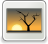# Regula falsi method for solving fuzzy nonlinear equation

Mustafa, Mamat and Fadhilah, Ahmad and Mohammed Ibrahim, Sulaiman (2016) Regula falsi method for solving fuzzy nonlinear equation. Far East Journal of Mathematical Sciences, 100 (6). pp. 873-884. ISSN 09720871Image FH02-FIK-16-06685.jpg Restricted to Registered users only Download (144kB)

## Abstract

In this paper, we introduce numerical method for solving algebraic fuzzy equation of degree n with fuzzy coefficients and crisp variable. The fuzzy quantities are presented in parametric form. Some numerical examples are provided to illustrate the efficiency of the method.

Item Type: Article Regula Falsi method, fuzzy nonlinear equation, triangular fuzzy numbers Q Science > QA Mathematics Faculty of Informatics & Computing Syahmi Manaf 13 Sep 2022 05:29 13 Sep 2022 05:29 http://eprints.unisza.edu.my/id/eprint/7566View Item